# MP Board Class 8th Maths Solutions Chapter 8 Comparing Quantities Ex 8.2

## MP Board Class 8th Maths Solutions Chapter 8 Comparing Quantities Ex 8.2

Question 1.
A man got a 10% increase in his salary. If his new salary is ₹ 1,54,000,find his original salary.
Solution:
We have,
Increase in salary = 10%
New salary = ₹ 154000
Let original salary be ₹ s.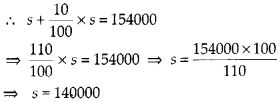∴ His original salary is ₹ 140000.

Question 2.
On Sunday 845 people went to the Zoo. On Monday only 169 people went. What is the percent decrease in the people visiting the Zoo on Monday?
Solution:
Number of people went to Zoo on Sunday = 845
Number of people went to Zoo on Monday = 169
Decrease in number of people = 845 – 169
= 676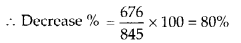Question 3.
A shopkeeper buys 80 articles for ₹ 2,400 and sells them for a profit of 16%. Find the selling price of one article.
Solution:
We have,
Cost price = ₹ 2400
Profit = 16%
Number of articles = 80∴ Selling price of 80 articlesThus, the selling price of one article = $$\frac{2784}{80}$$
= ₹ 34.80

Question 4.
The cost of an article was ₹ 15,500. ₹ 450 were spent on its repairs. If it is sold for a profit of 15%, find the selling price of the article.
Solution:
Cost of an article = ₹ 15500
Money spent on repairs = ₹ 450
∴ Total cost = Cost + Repairs = ₹ 15950
Profit = 15 %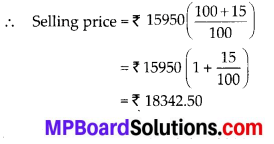Question 5.
A VCR and TV were bought for ? 8000 each. The shopkeeper made a loss of 4% on the VCR and a profit of 8% on the TV. Find the gain or loss percent on the whole transaction.
Solution:
Cost of VCR = ₹ 8000
Cost of TV = ₹ 8000
Total cost = 8000 + 8000 = ₹ 16000
Loss on VCR = 4 %Question 6.
During a sale, a shop offered a discount of 10% on the marked prices of all the items. What would a customer have to pay for a pair of jeans marked at ₹ 1450 and two shirts marked at ₹ 850 each?
Solution:
We have,
Cost of a pair of jeans = ₹ 1450
Cost of two shirts = 2 × 850 = ₹ 1700
∴ Total cost = 1450 + 1700 = ₹ 3150
Discount = 10 %Question 7.
A milkman sold two of his buffaloes for ₹ 20,000 each. On one he made a gain of 5% and on the other a loss of 10%. Find his overall gain or loss. (Hint: Find C.P. of each).
Solution:
We have,
Selling price of each buffalo = ₹ 20000
Total selling price = ₹ 40000
On buffalo 1, profit = 5 %
Cost price of buffalo 1Total cost price = 19047.62 + 22222.22
= ₹ 41269.84
Since, cost price is greater than the selling price.
∴ Loss = 41269.84 – 40000 = ₹ 1269.84

Question 8.
The price of a TV is ₹ 13000. The sales tax charged on it is at the rate of 12%. Find the amount that Vinod will have to pay if he buys it.
Solution:
Cost price of TV = ₹ 13000
Sales tax = 12 %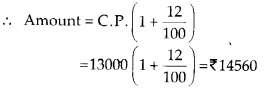Question 9.
Arun bought a pair of skates at a sale where the discount given was 20%. If the amount he pays is ₹ 1,600, find the marked price.
Solution:
Selling price = ₹ 1600
Discount = 20 %
We know,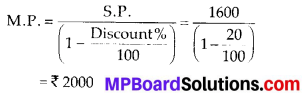Question 10.
I purchased a hair-dryer for ? 5,400 including 8% VAT. Find the price before VAT was added.
Solution:
Cost price = ₹ 5400
VAT = 8%